# Percentages

Go back to  'Ratio, Proportion, Percentages'

The grid given below is made of 100 blocks. Each block has a length of breadth of 1 unit.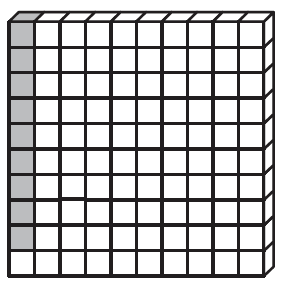In the grid given above 9 blocks out of 100 are shaded. In fractional form it can be written as 9/100. Percent means “out of 100”. If 20 out of 100 boxes are shaded, then we say that 20 percent or 20% of the boxes are shaded.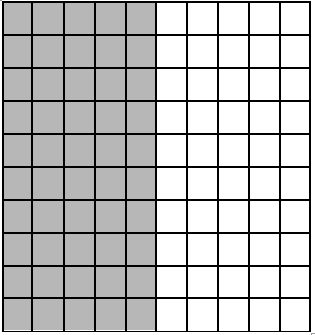In the picture given above 50 blocks out of 100 are shaded, we can also say that 50 percent or 50% of the boxes are shaded.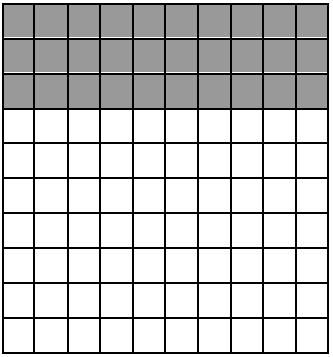In the picture given above 50 blocks out of 100 are shaded, we can also say that 50 percent or 50% of the boxes are shaded.

## Relationship between fractions and percentages

-    A percentage can also be expressed in fractional or decimal form.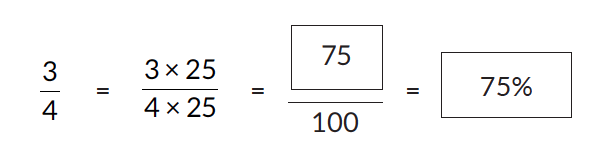In the example given above ¾ can also be written as 75% or 0.75.

-    A fraction can also be converted to a percentage.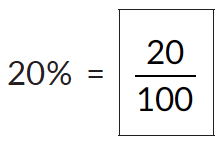Converting it to its lowest form would be,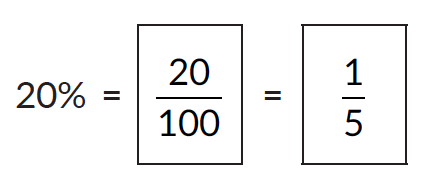## How to calculate percentages?

1. Let’s consider an example. Let’s say we want to calculate what percentage of 50 is 13.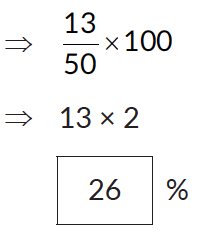2. Let’s take another example to understand how to calculate the value of a given percentage from the whole.

\begin{align}20\%\;\text{ of } \; 80 = {20\over 100} \times 80 = 16\end{align}

3. Let’s take another example find the whole whose 20% is 50.

We need to find the number whose 20% or \begin{align}1\over 5\end{align} th is 50. Here is a strip. 50 is the  \begin{align}1\over 5\end{align}th value of the whole strip.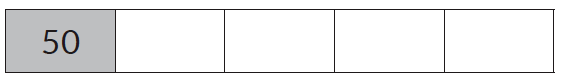Because the strip's been divided into equal parts, the value of every other part is also 50.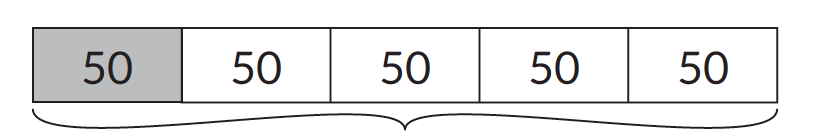This means the value of the strip is 250.

It can also be visualized as,

\begin{align} {20\over 100} \times ? &= 50 \\ &= 50 \times {100 \over 20} \\ &= 250 \end{align}

## An introduction to percentage change

Percent increase or decrease are measures of percent change. It shows the extent to which something has gained or lost value. It is an indicator of positive or negative delta. Percent changes are useful to help people understand changes in a value over time. Let’s try and understand this using an example,

### Increase in percentage

Joey weighed 50 kg in March and 55 kg a month later. Find the percentage increase in his weight.

Joey’s weight in March = 50 Kg
Joey’s weight in April = 55 kg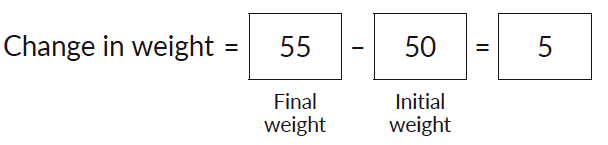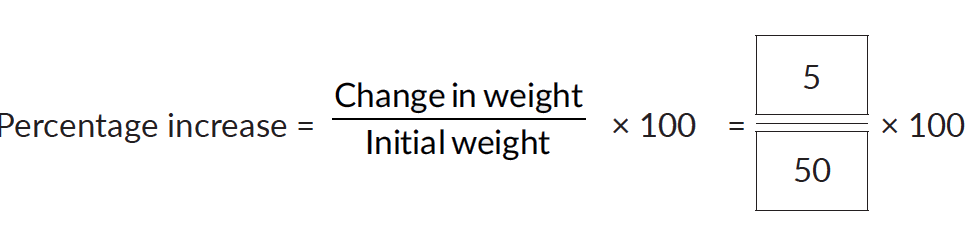Percentage increase = 10%

### Decrease in percentage

If 240 is decreased to 180, find the percentage decrease.

Change = 240 - 180 = 60

Percentage decrease \begin{align} = {60\over 240} \times 100 = 25\% \end{align}

## Calculation of successive changes in percentages

Successive changes in percentage is about two or more percentage changes on a given value. Let’s try and understand this using an example.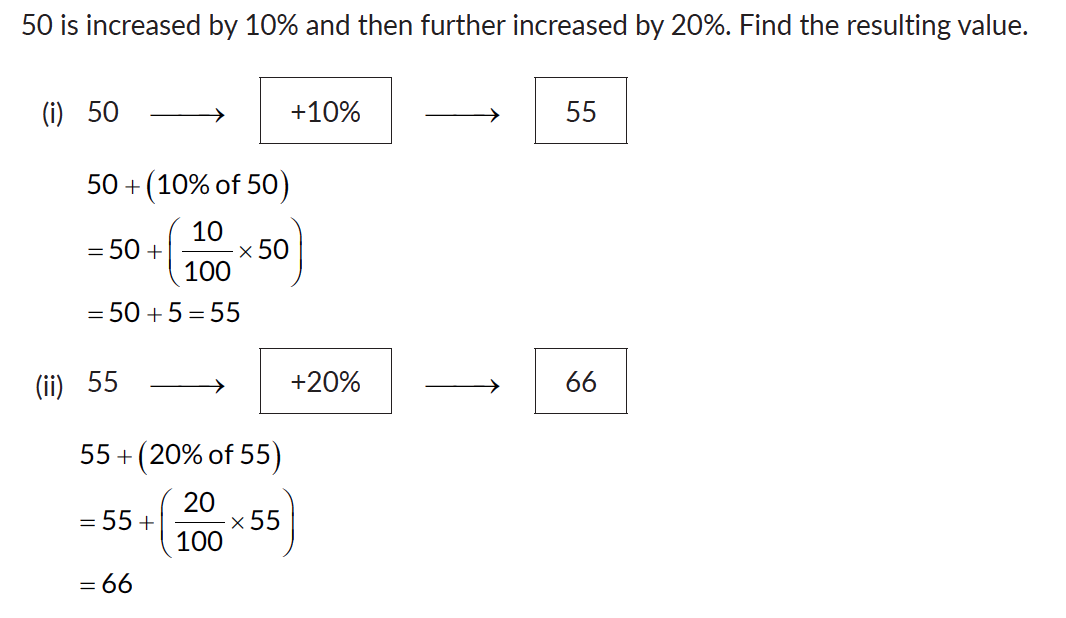## Tips and Tricks

• Remember some easy percentages. Use them to estimate or calculate other percentages.

50% is half. So, simply divide the number by 2.
25% is a quarter. Simply divide the number by 4.
20% is a fifth. Divide the number by 5.
10% is one-tenth. So, simply divide the number by 10. That is, move the decimal one place to the left.
1% is one-hundredth. So, either divide by 100 or move the decimal two places to the left.

• With this, you’re ready to calculate any percentage. To find 60%, first find 50% then find 10% and add the two.

To find 63% find 60% as described earlier. Then find 1% and add it thrice to 60%.

• To calculate 90%, it may be easier to calculate 10% and subtract that from the original number. E.g. 90% of 32? 10% of 32 is 3.2. Subtract this from 32 to get 90% of 32 = 28.8
• x% of y is the same as y% of x.

If asked to find 16% of 25 you can get this easily by finding 25% of 16… that is one-fourth of 16 = 4. The latter is much easier to do.

• Remember percentages can be greater than 100% too. For instance, 125% of a number is that number + 25% of it

So, 125% of 36 will be 36 + 9 = 45 (the 9 comes from dividing 36 by 4 to find its quarter).